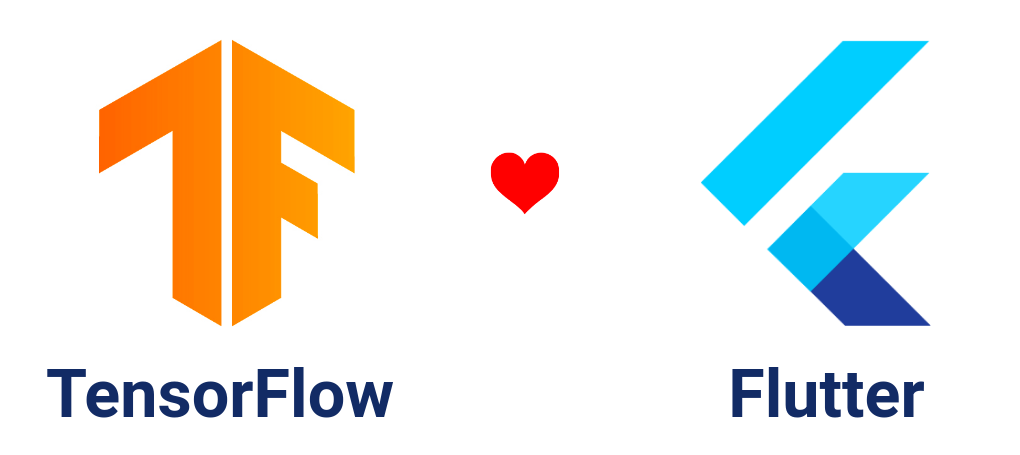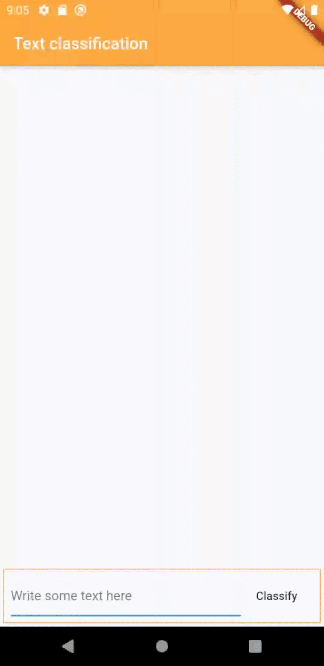## 在 Flutter 中使用 TensorFlow Lite 插件实现文字分类tflite_flutter 插件的核心特性：

• 它提供了与 TFLite Java 和 Swift API 相似的 Dart API，所以其灵活性和在这些平台上的效果是完全一样的
• 通过 dart:ffi 直接与 TensorFlow Lite C API 相绑定，所以它比其它平台集成方式更加高效。
• 无需编写特定平台的代码。
• 通过 NNAPI 提供加速支持，在 Android 上使用 GPU Delegate，在 iOS 上使用 Metal Delegate。

## 初始化配置

### Linux 和 Mac 用户

install.sh 拷贝到您应用的根目录，然后在根目录执行 sh install.sh，本例中就是目录 text_classification_app/

### Windows 用户

install.bat 文件拷贝到应用根目录，并在根目录运行批处理文件 install.bat，本例中就是目录 text_classification_app/

## 获取插件

pubspec.yaml 添加 tflite_flutter: ^<latest_version>详情）。

## 下载模型

text_classification.tflitetext_classification_vocab.txt 文件拷贝到 text_classification_app/assets/ 目录下。

pubspec.yaml 文件中添加 assets/

assets:
- assets/


## 预处理

1. 对段落文本进行分词，然后使用预定义的词汇集将它转换为一组词汇 ID；
2. 将生成的这组词汇 ID 输入 TensorFlow Lite 模型里；
3. 从模型的输出里获取当前段落是积极或者是消极的概率值。

import 'package:flutter/services.dart';

class Classifier {
final _vocabFile = 'text_classification_vocab.txt';

Map<String, int> _dict;

Classifier() {
}

final vocab = await rootBundle.loadString('assets/$_vocabFile'); var dict = <String, int>{}; final vocabList = vocab.split('\n'); for (var i = 0; i < vocabList.length; i++) { var entry = vocabList[i].trim().split(' '); dict[entry] = int.parse(entry); } _dict = dict; print('Dictionary loaded successfully'); } }  加载字典 现在我们来编写一个函数对原始字符串进行分词。 import 'package:flutter/services.dart'; class Classifier { final _vocabFile = 'text_classification_vocab.txt'; // 单句的最大长度 final int _sentenceLen = 256; final String start = '<START>'; final String pad = '<PAD>'; final String unk = '<UNKNOWN>'; Map<String, int> _dict; List<List<double>> tokenizeInputText(String text) { // 使用空格进行分词 final toks = text.split(' '); // 创建一个列表，它的长度等于 _sentenceLen，并且使用 <pad> 的对应的字典值来填充 var vec = List<double>.filled(_sentenceLen, _dict[pad].toDouble()); var index = 0; if (_dict.containsKey(start)) { vec[index++] = _dict[start].toDouble(); } // 对于句子里的每个单词在 dict 里找到相应的 index 值 for (var tok in toks) { if (index > _sentenceLen) { break; } vec[index++] = _dict.containsKey(tok) ? _dict[tok].toDouble() : _dict[unk].toDouble(); } // 按照我们的解释器输入 tensor 所需的形状 [1,256] 返回 List<List<double>> return [vec]; } }  ## 使用 tflite_flutter 进行分析 这是本文的主体部分，这里我们会讨论 tflite_flutter 插件的用途。 这里的分析是指基于输入数据在设备上使用 TensorFlow Lite 模型的处理过程。要使用 TensorFlow Lite 模型进行分析，需要通过 解释器 来运行它，了解更多 创建解释器，加载模型 tflite_flutter 提供了一个方法直接通过资源创建解释器。 static Future<Interpreter> fromAsset(String assetName, {InterpreterOptions options})  由于我们的模型在 assets/ 文件夹下，需要使用上面的方法来创建解析器。对于 InterpreterOptions 的相关说明，请 参考这里 import 'package:flutter/services.dart'; // 引入 tflite_flutter import 'package:tflite_flutter/tflite_flutter.dart'; class Classifier { // 模型文件的名称 final _modelFile = 'text_classification.tflite'; // TensorFlow Lite 解释器对象 Interpreter _interpreter; Classifier() { // 当分类器初始化以后加载模型 _loadModel(); } void _loadModel() async { // 使用 Interpreter.fromAsset 创建解释器 _interpreter = await Interpreter.fromAsset(_modelFile); print('Interpreter loaded successfully'); } }  创建解释器的代码 如果您不希望将模型放在 assets/ 目录下，tflite_flutter 还提供了工厂构造函数创建解释器，更多信息 我们开始进行分析！ 现在用下面方法启动分析： void run(Object input, Object output);  注意这里的方法和 Java API 中的是一样的。 Object inputObject output 必须是和 Input Tensor 与 Output Tensor 维度相同的列表。 要查看 input tensors 和 output tensors 的维度，可以使用如下代码： _interpreter.allocateTensors(); // 打印 input tensor 列表 print(_interpreter.getInputTensors()); // 打印 output tensor 列表 print(_interpreter.getOutputTensors());  在本例中 text_classification 模型的输出如下： InputTensorList: [Tensor{_tensor: Pointer<TfLiteTensor>: address=0xbffcf280, name: embedding_input, type: TfLiteType.float32, shape: [1, 256], data: 1024] OutputTensorList: [Tensor{_tensor: Pointer<TfLiteTensor>: address=0xbffcf140, name: dense_1/Softmax, type: TfLiteType.float32, shape: [1, 2], data: 8]  现在，我们实现分类方法，该方法返回值为 1 表示积极，返回值为 0 表示消极。 int classify(String rawText) { // tokenizeInputText 返回形状为 [1, 256] 的 List<List<double>> List<List<double>> input = tokenizeInputText(rawText); // [1,2] 形状的输出 var output = List<double>(2).reshape([1, 2]); // run 方法会运行分析并且存储输出的值 _interpreter.run(input, output); var result = 0; // 如果输出中第一个元素的值比第二个大，那么句子就是消极的 if ((output as double) > (output as double)) { result = 0; } else { result = 1; } return result; }  用于分析的代码 在 tflite_flutter 的 extension ListShape on List 下面定义了一些使用的扩展： // 将提供的列表进行矩阵变形，输入参数为元素总数 // 保持相等 // 用法：List(400).reshape([2,10,20]) // 返回 List<dynamic> List reshape(List<int> shape) // 返回列表的形状 List<int> get shape // 返回列表任意形状的元素数量 int get computeNumElements  最终的 classifier.dart 应该是这样的： import 'package:flutter/services.dart'; // 引入 tflite_flutter import 'package:tflite_flutter/tflite_flutter.dart'; class Classifier { // 模型文件的名称 final _modelFile = 'text_classification.tflite'; final _vocabFile = 'text_classification_vocab.txt'; // 语句的最大长度 final int _sentenceLen = 256; final String start = '<START>'; final String pad = '<PAD>'; final String unk = '<UNKNOWN>'; Map<String, int> _dict; // TensorFlow Lite 解释器对象 Interpreter _interpreter; Classifier() { // 当分类器初始化的时候加载模型 _loadModel(); _loadDictionary(); } void _loadModel() async { // 使用 Intepreter.fromAsset 创建解析器 _interpreter = await Interpreter.fromAsset(_modelFile); print('Interpreter loaded successfully'); } void _loadDictionary() async { final vocab = await rootBundle.loadString('assets/$_vocabFile');
var dict = <String, int>{};
final vocabList = vocab.split('\n');
for (var i = 0; i < vocabList.length; i++) {
var entry = vocabList[i].trim().split(' ');
dict[entry] = int.parse(entry);
}
_dict = dict;
}

int classify(String rawText) {
// tokenizeInputText  返回形状为 [1, 256] 的 List<List<double>>
List<List<double>> input = tokenizeInputText(rawText);

//输出形状为 [1, 2] 的矩阵
var output = List<double>(2).reshape([1, 2]);

// run 方法会运行分析并且将结果存储在 output 中。
_interpreter.run(input, output);

var result = 0;
// 如果第一个元素的输出比第二个大，那么当前语句是消极的

if ((output as double) > (output as double)) {
result = 0;
} else {
result = 1;
}
return result;
}

List<List<double>> tokenizeInputText(String text) {
// 用空格分词
final toks = text.split(' ');

var index = 0;
if (_dict.containsKey(start)) {
vec[index++] = _dict[start].toDouble();
}

// 对于句子中的每个单词，在 dict 中找到相应的 index 值
for (var tok in toks) {
if (index > _sentenceLen) {
break;
}
vec[index++] = _dict.containsKey(tok)
? _dict[tok].toDouble()
: _dict[unk].toDouble();
}

// 按照我们的解释器输入 tensor 所需的形状 [1,256] 返回 List<List<double>>
return [vec];
}
}



// 创建 Classifier 对象
Classifer _classifier = Classifier();
// 将目标语句作为参数，调用 classify 方法
_classifier.classify("I liked the movie");
// 返回 1 （积极的）
_classifier.classify("I didn't liked the movie");
// 返回 0 （消极的）## 答疑

#### 问：tflite_flutter 和 tflite v1.0.5 有哪些区别？

tflite v1.0.5 侧重于为特定用途的应用场景提供高级特性，比如图片分类、物体检测等等。而新的 tflite_flutter 则提供了与 Java API 相同的特性和灵活性，而且可以用于任何 tflite 模型中，它还支持 delegate。

#### 问：如何使用 tflite_flutter 创建图片分类应用？有没有类似 TensorFlow Lite Android Support Library 的依赖包？

TensorFlow Lite Flutter Helper Library 为处理和控制输入及输出的 TFLite 模型提供了易用的架构。它的 API 设计和文档与 TensorFlow Lite Android Support Library 是一样的。更多信息请 参考这里

## 致谢

• 译者：Yuan，谷创字幕组
• 审校：Xinlei、Lynn Wang、Alex，CFUG 社区。

posted on 2020-09-16 10:43  Flutter社区  阅读(68)  评论(0编辑  收藏